Next: Vortex Sheets Up: Incompressible Aerodynamics Previous: Cylindrical Airfoils

# Zhukovskii's Hypothesis

According to the previous analysis, the lift acting on a cylindrical airfoil of elliptic cross-section, situated in a uniform, high Reynolds number wind, depends on the circulation,, of air about the airfoil. But, how can we determine the value of this circulation?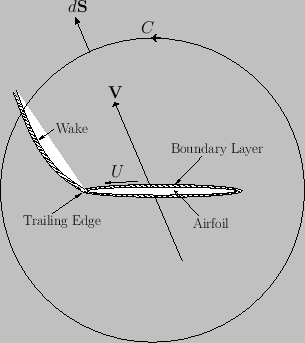Figure 9.3 shows the boundary layer and wake of a streamlined airfoil. The boundary layer, which is localized on the surface of the airfoil, has a vortex intensity per unit length in the-direction equal to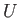, whereis the tangential air speed immediately above the layer. [See Equation (9.64).] Moreover, the wake is emitted by the airfoil's trailing edge, and subsequently convected by the external air flow. (See Section 8.6.) Note that the flow is irrotational everywhere apart from inside the boundary layer and the wake. According to the analysis of Section 5.8, the rate of change of the circulation,, around some curvethat encloses the airfoil is equal to minus the flux of-directed vorticity across this curve: that is,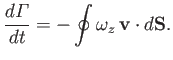(9.36)

Here,is the wind velocity,the wind vorticity, and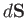an outward surface element (of unit depth in the-direction) lying on. We expect the vorticity flux to be independent of the size and shape of, otherwise the circulation of the flow,, about the airfoil would not have a unique value. In the limit thatbecomes very large,, whereis the incident wind velocity. Thus,(9.37)

whereis the wind speed, andthe vortex intensity per unit length in the wake (at the point where it crosses the curve). Here, we are assuming that the vorticity within the wake is convected by the flow, giving rise to a net flux of vorticity across. Because the wake is essentially an extension of the boundary layer, it is reasonable to assume that its vortex intensity per unit length is proportional to that in the boundary layer at the airfoil's trailing edge, where the wake and boundary layer intersect. In other words,, whereis the tangential velocity immediately above the trailing edge of the airfoil, and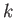is a constant. It follows that(9.38)

According to Equation (9.27), the tangential velocity just above the surface of the airfoil is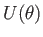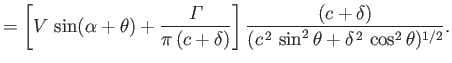(9.39)

Hence, given that the airfoil's trailing edge corresponds to, we obtain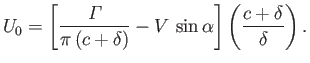(9.40)

Thus, Equation (9.38) yields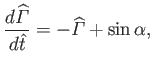(9.41)

where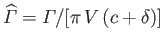,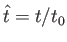, and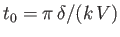. Assuming that the circulation of the flow about the airfoil is initially zero (i.e.,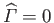at), the previous equation can be solved to give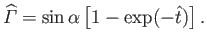(9.42)

Clearly, asthe normalized circulation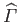asymptotes to the constant value(9.43)

The corresponding constant value of the unnormalized circulation is(9.44)

According to Equation (9.40), when the circulation,, takes the value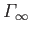the tangential velocity at the airfoil's training edge,, is zero. In other words, the steady-state circulation set up around the airfoil is such as to render its trailing edge a stagnation point of the flow. This conclusion is known as Zhukovskii's hypothesis, after its discoverer N.E. Zhukovskii (1847-1921).Incidentally, it should be clear, from the previous discussion, that the air circulation about the airfoil is only able to change its value because of the presence of the boundary layer, and the associated wake that trails from the airfoil's trailing edge. This follows because the flow is irrotational everywhere except within the boundary layer and the wake. Moreover, as we have seen, a change in circulation is necessarily associated with a net vorticity flux away from the airfoil, and such a flux cannot be carried by an irrotational wind. Thus, in the absence of the boundary layer and the wake, the air circulation about the airfoil would be constrained to remain zero (assuming that it was initially zero), in accordance with the Kelvin circulation theorem. (See Section 5.8.) This implies, from Equation (9.31), that zero lift would act on the airfoil, irrespective of its shape, and irrespective of the incident wind speed or direction. In other words, flight would be impossible. Fortunately, as long as the tangential air velocity at the trailing edge of the airfoil is non-zero, the wake that trails behind the airfoil carries a net flux of-directed vorticity, which causes the airfoil circulation to evolve in time. This process continues until the circulation becomes such that the tangential velocity at the airfoil's trailing edge is zero: that is, such that the trailing edge is a stagnation point. Thereafter, the circulation remains constant (assuming that the wind speed and direction remain constant). Figures 9.4 and 9.5 show the streamlines of the flow around a slender cylindrical airfoil of elliptic cross-section situated in a uniform, high Reynolds number wind whose direction of incidence is slightly inclined to the airfoil's major axis. In the first figure, the air circulation about the airfoil is zero. In the second figure, the circulation is such as to make the trailing edge of the airfoil a stagnation point.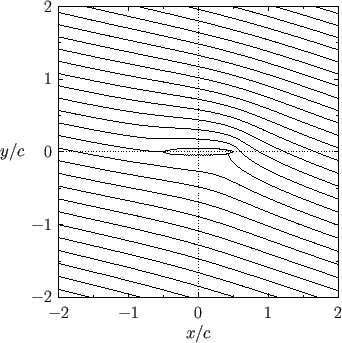According to Equations (9.31) and (9.44), when the air circulation about the airfoil has attained its steady-state value,, the lift acting on the airfoil becomes(9.45)

The lift is positive (i.e., upward) when(i.e., when the wind is incident on the airfoil's bottom surface), negative (i.e., downward) when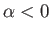(i.e., when the wind is incident on the airfoil's top surface), and zero when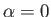(i.e., when the wind is incident parallel to airfoil's major axis). Incidentally, the angle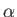is conventionally termed the angle of attack. Finally, from Equations (9.35) and (9.43), the focus of the airfoil is located a distance(9.46)

in front of the centroid of its cross-section. In the limit that the airfoil becomes very thin (i.e.,), this distance asymptotes to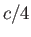. Thus, we conclude that the focus of a thin airfoil, which is defined as the point of action of the lift, is located one quarter of the way along the airfoil from its leading edge.The previous analysis is premised on the assumption that there is no appreciable separation of the boundary layer from the back of the airfoil, which implies the neglect of form drag. We can check that this assumption is reasonable by calculating the approximate locations of the boundary layer separation points using the analysis of Section 8.10. Let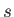represent arc-length along the surface of the airfoil, measured from the front stagnation point. Assuming that, in accordance with Zhukovskii's hypothesis, the circulation is such that, this stagnation point is located at, where. [See Equation (9.49).] It follows that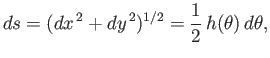(9.47)

where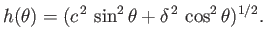(9.48)

Moreover, from Equations (9.39) and (9.44), the tangential air speed just above the surface of the airfoil can be written(9.49)

with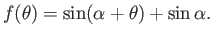(9.50)

In addition, it can be shown that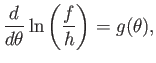(9.51)

where(9.52)

According to the analysis of Section 8.10, the separation points are located at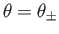, where, and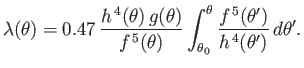(9.53)

Here,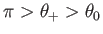and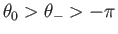. Moreover, the- and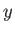-coordinates of the separation points are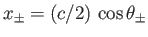and, respectively. Figure 9.6 shows the angular locations of the separation points, calculated as a function of the angle of attack, for cylindrical airfoils of various different ellipticity,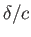. (Note that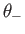has been re-expressed as an angle in the range 0 to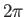.) It can be seen that for a bluff airfoil (e.g.,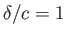) the angular distance between the separation points is large, indicating the presence of a wide wake, and a high associated form drag (because the magnitude of form drag is roughly proportional to the width of the wake). On the other hand, for a slender airfoil (e.g.,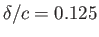) the angular distance between the separation points is much smaller, indicating the presence of a narrow wake, and a low associated form drag. However, in the latter case, as the angle of attack is gradually increased from zero, there is an initial gradual increase in the angular distance between the separation points, followed by an abrupt, and very large, increase. We would expect there to be a similar gradual increase in the form drag, followed by an abrupt, and very large, increase. The value of the angle of attack at which this abrupt increase occurs is termed the critical angle of attack. We conclude that the previous analysis, which neglects form drag, is valid only for slender airfoils whose angles of attack do not exceed the critical value (which is generally only a few degrees).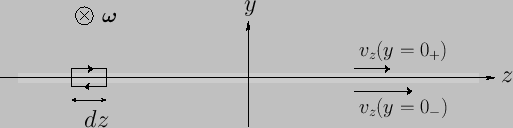Next: Vortex Sheets Up: Incompressible Aerodynamics Previous: Cylindrical Airfoils
Richard Fitzpatrick 2016-03-31